# Grade - math word problems

#### Number of problems found: 5417

• Tractor wheels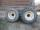Smaller tractor wheel has a circumference of 3 meters, bigger 4 meters. On what path executes smaller wheel 6 turns over more bigger?
• Reducing balance method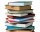A company buys an item having a useful life of 10 years for 1,000,000. If the company depreciates the item by the reducing balance method, a. Determine the depreciation for the first year. b. Estimate the depreciation for the second and third years. c. Wh
• Five pumpsThe water tank is filled with two pumps in 48 minutes. How long would it take to fill it with 5 same pumps?
• Sum on diceWe have two dice. What is the greater likelihood of fall a total sum 7 or 8 ? (write 7, 8 or 0 if the probabilities are the same)?
• Where and when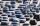The truck left Kremnica at 11:00 h at a speed of 60km/h. At 12:30 h, the passenger car started at an average speed of 80km/h. How many kilometers from Kremnica will the passenger car reach truck, and when?
• Secret treasureScouts have a tent in the shape of a regular quadrilateral pyramid with a side of the base 4 m and a height of 3 m. Determine the radius r (and height h) of the container so that they can hide the largest possible treasure.
• Hypotenuse - RTA triangle has a hypotenuse of 55 and an altitude to the hypotenuse of 33. What is the area of the triangle?
• Guess a fractionTom was asked to guess a fraction. The sum of 1/2 the numerator and 1/3 of its denominator is 30. If Tom subtracts 36 from its denominator, the fraction becomes 1/3. What is the fraction that Tom was asked to guess? (Leave your answer in simplest form)
• The lowestThe lowest temperature on Thursday was 20°C. The lowest temperature on Saturday was 12°C. What was the difference between the lowest temperatures?
• Fishing boat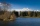The fishing boat caught 14 fish in one day. How many fish will catch 4 fishing boats in 8 days?
• A boyA boy of height 1.7m is standing 30m away from flag staff on the same level ground . He observes that the angle of deviation of the top of flag staff is 30 degree. Calculate the height of flag staff.
• EmployeesOf all 360 employees, there are 11/12 women. How many men work in a company?
• Hectares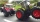The tractor plows the first day of 4.5ha, the second day 6.3ha and the third day 5.4ha. It worked whole hours a day, and its hourly performance did not change and was the highest of the possible. How many hectares did it plow in one hour (what is it perfo
• A boxA box is 15 centimeters long, 4 centimeters wide, and 3 centimeters tall what is the diagonal S of the bottom side? What is the length of the body diagnol R?
• Products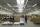15 products are 48 € more expensive than 12 products. How many euros will it cost five same products?
• Coffee shop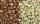To the coffee shop brought 2 types of coffee totally 50 kg. The first type was CZK 220 per kilogram, coffee second type 300 CZK per 1 kg. For all the coffee trader earned CZK 12,000. How many kilograms of coffee of first type and how many kilograms of co
• Isosceles triangleThe circumference of the isosceles triangle is 32.5 dm. Base length is 153 cm. How long is the leg of this triangle?
• Long bridge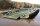Roman walked on the bridge. When he heard the whistle, he turned and saw running Kamil at the beginning of the bridge. If he went to him, they would meet in the middle of the bridge. Roman, however, rushed and so did not want to waste time returning 150m.
• ParallelogramCalculate the missing side of a parallelogram, if you know the perimeter o and one side: a) o = 7.2 cm; b = 1.8 cm b) o = 5.4 cm; a = 1.9 cm
• Sale offProduct cost 95 euros before sale off. After sale off cost 74 euros and 10 cents. About what percentage of produt became cheaper?

Do you have an interesting mathematical word problem that you can't solve it? Submit a math problem, and we can try to solve it.

We will send a solution to your e-mail address. Solved examples are also published here. Please enter the e-mail correctly and check whether you don't have a full mailbox.

Please do not submit problems from current active competitions such as Mathematical Olympiad, correspondence seminars etc...